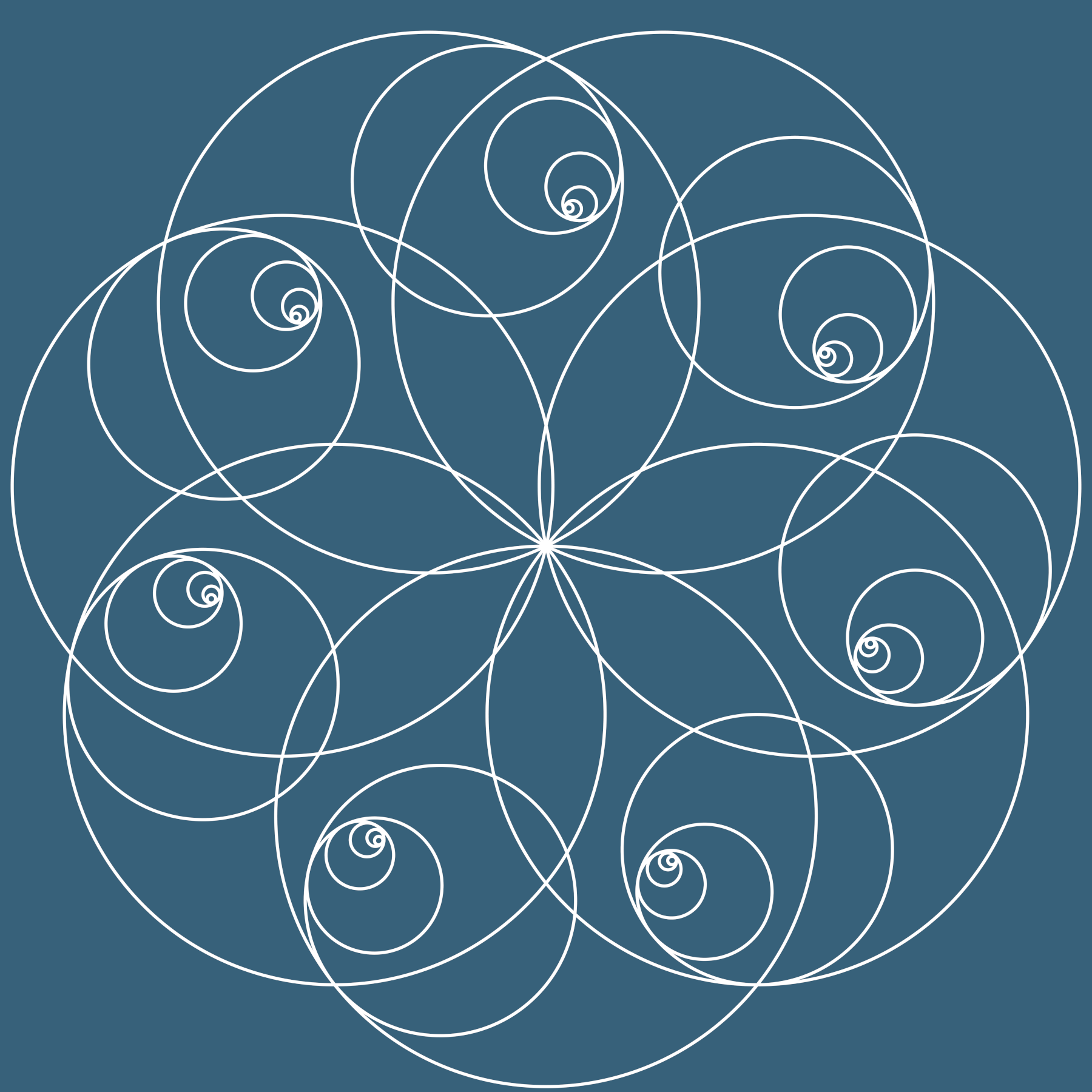Convergent Gershgorin Disks Digital Print 2018

The cover art was inspired by the article in this issue by Melman. It imagines a series of $7\times 7$ similar matrices having full rank, where the Gershgorin disks become smaller and smaller, converging on the eigenvalues. Given a square $n \times n$ matrix $A$ of rank $n$, it can be diagonlized to a matrix $D$; thus the matrices $A$ and $D$ are similar. If one applies the Gershgorin circle theorem (GCT) to $D$, one will just get circles of radius 0 (thus points) at the eigenvalues. On the other hand, the GCT applied to $A$ will generally yield circles of various size containing the eigenvalues. Any matrix $B$ similar to $A$ (and $D$) will have the same eigenvalues, so the GCT applied to $B$ will give another set of circles around the eigenvalues.